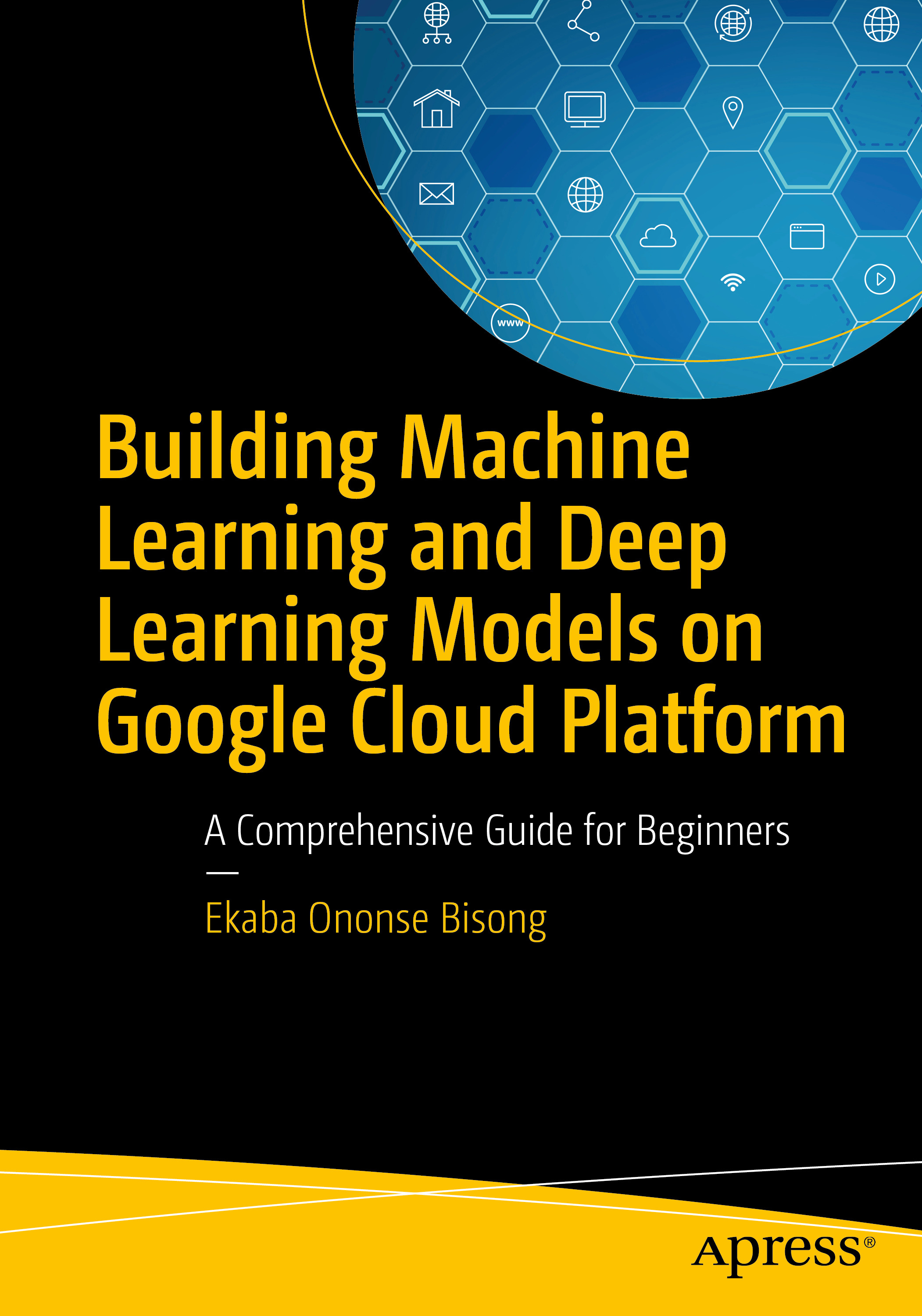Welcome to the seminar series on Building Machine Learning and Deep Learning Models on Google Cloud Platform. This goal of this seminar is to inform, educate and generate interest in the field of analytics, machine learning and deep learning which is termed data science from an industry perspective.

This seminar is divided into four parts, covering:
• An Introduction to Google Cloud Platform Infrastructure and Services
• An Introduction to Programming for Data Science
• An Introduction to Machine Learning
• An Introduction to Deep Learning

This seminar is by no means exhaustive. However, it presents just enough to give a solid conceptual understanding of what these technologies are all about, and how they are currently revolutionizing various sectors. After going through this seminar series, a complete beginner can have a good background to delve deeper and understand more.

Moreso, the contents of this material forms part of my upcoming book with Apress with the same title.Part 1: Google Cloud Platform Infrastructure and Services
Computational Challenges of Training Learning Models, The Cloud to the Rescue, Enter Google Cloud Platform
An Overview of Google Cloud Platform Services
Cloud Compute, Cloud Storage, Big Data/ Analytics, Cloud AI, Skinny Data Migration Pipeline
Setting Up an account on Google Cloud Platform
Creating an Account, GCP Resources: Projects, Accessing Cloud Platform Services, Account Users and Permissions, The Cloud Shell
Create a bucket, Uploading data to a bucket, Delete objects from a bucket, Free up storage resource
Enable Google Compute Engine and Cloud Source Repositories APIs, Launch a Datalab Instance from gcloud, Open a notebook, Shutting down/ Deleting the instance
Part 2: Programming for Data Science
Introduction to Python Programming
Data and Operations, Data Types, Arithmetic and Boolean Operations, The print() statement, Control Structures, Functions, Packages and Modules
Numpy
NumPy 1-D Array, NumPy Datatypes, Indexing + Fancy Indexing (1-D), Slicing a 1-D Array, Basic Math Operations on Arrays: Universal Functions, Higher-Dimensional Arrays, Creating 2-D arrays (Matrices), Creating 3-D arrays, Indexing/ Slicing of Matrices, Matrix Operations: Linear Algebra, Reshaping, Broadcasting, Loading Data
Pandas
Pandas Datastructures, Data Indexing (Selection/ Subsets), DataFrame Manipulation, Handling Missing Data, Data Aggregation (Grouping), Statistical Summaries, Importing Data
Matplotlib and Seaborn
Matplotlib vs. Seaborn, Pandas plotting methods, Univariate plots: Line plot, Bar plot, Histogram/ Density plots, Box and whisker plots, Multivariate plots: Scatter plot, Pairwise scatter plots, Correlation matrix plots
Part 3: Machine Learning
What is Machine Learning
The Role of Data, Core Components of Machine Learning, Training, Test and Validation datasets, Bias vs. Variance Tradeoff, Evaluating Model Quality, Resampling Techniques, Machine learning algorithms, Machine Learning Packages
Linear Regression
A visual representation, Optimization for Machine Learning: Gradient Descent, Cost Function for Linear Regression, What are its strengths and weaknesses
Logistic Regression
The Logit or Sigmoid Model, The Logistic Regression Cost Function, Multi-class classification/ multinomial logistic regression
Scikit-learn
Loading Sample datasets from Scikit-learn, Splitting the Dataset into training and test sets, Data Preprocessing, Machine learning algorithms, Feature Engineering, Resampling Methods, Model evaluation, Pipelines: Streamlining Machine Learning Workflows, Model tuning
Part 4: Deep Learning
Deep Learning Explained: Artificial Neural Networks
The representation challenge, An Inspiration from the Brain, The Neural Network Architecture, Training the Network, Cost Function or Loss Function, The Backpropagation Algorithm, Activation Functions
Convolutional Neural Networks
An Overview of Convolutional Neural Networks, Local Receptive Fields of the Visual Cortex, CNN Advantage Over MLP, Convolutional Layer, Pooling Layer, Fully Connected Layer
Recurrent Neural Networks
An Overview of RNN, The Recurrent Neuron, Unfolding the Recurrent Computational Graph, Basic Recurrent Neural Network, Recurrent Connection Schemes, Training the Recurrent Network: Backpropagation Through Time, The Long Short Term Memory (LSTM) Network
TensorFlow
The case for Computational Graphs, Navigating through the TensorFlow API, TensorBoard, The Low/Mid-Level API: Building Computational Graphs and Sessions, Linear Regression with TensorFlow, Classification with TensorFlow, Multilayer Perceptron (MLP), Running TensorFlow with GPUs, Convolutional Neural Networks, Recurrent Neural Networks, Autoencoders, Building Efficient Input Pipelines with the Dataset API, TensorFlow High-Level APIs: Using Estimators
Keras
The Anatomy of a Keras Program, Multilayer Perceptron (MLP) with Keras, Using the Dataset API with tf.keras, Model Visualization with Keras, TensorBoard with Keras, Checkpointing to Select Best Models, Convolutional Neural Networks (CNNs) with Keras, Recurrent Neural Networks (RNNs) with Keras, Stacked LSTM, Long-term Recurrent Convolutional Network (CNN LSTM) Encoder-Decoder LSTM, Bidirectional LSTM